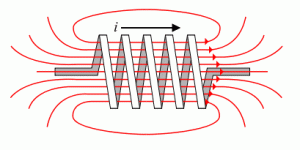# Inductance Definition

An inductor has the property to oppose any change in the current passing through it. This property is called inductance. When a current flows through a conductor, a magnetic field is created.

## Magnetic field lines & Counter electromotive force (CEMF)

Lines of force of the magnetic field expands starting in the center of the electrical conductor and then moving away.

First the lines of force pass through the conductor itself and then through the air. While these lines of force are in the electrical conductor, an electromotive force (emf) is generated in the conductor itself. The voltage generated has an opposite direction of the electric current. Because of this, the force is called: Counter-electromotive force (CEMF).By inductiveload (Own drawing, done in Inkscape 0.44) [Public domain], via Wikimedia Commons

This effect causes that temporarily the maximum value of current in the conductor is not reached. When, eventually, the current variation disappears (the current has a constant value), the lines of force no longer expand and the counter electromotive force disappears.

When current begins to flow through the conductor, the lines of force of the magnetic field begin to expand rapidly and a large counter electromotive force is achieved. At this time the counter electromotive force is almost equal to the applied voltage source. Thus, the voltage source and the counter electromotive force almost cancel each other and the current flow is small.

After some time, the lines of magnetic field reaches its maximum value, the Counter electromotive force (CEMF) no longer is generated and the only electromotive force is the voltage source. At this time, the maximum current flows on the circuit because there is no opposition of the inductance.

This property of opposing the current changes, self-inducing an electromotive force in the opposite direction (counter electromotive force) is called inductance. The unit of inductance is the henry (H) and is represented by the capital letter “L”.

## What is the inductance of a coil?

L = VL/(di/dt)

where:

• L is the inductance in Henries
• VL is the voltage across the coil in Volts
• di/dt is the rate of change of current in Amperes per second, A/s

By increasing the number turns within a coil, we can increase the coils inductance. The relationship between inductance (self inductance) and tne number of turns is:

L = NΦ/I

where:

• L is the inductance in Henries
• N is the number of turns
• Φ is the magnetic flux in Webers (Wb)
• I is the current in Amperes

Example # 1

What is the self-induced EMF produced in a 200 mH coil when passing a DC current of 3 Amps after a time of 20 ms?

• L = 200 mH = 0.2 Henries
• I = 3 Amps
• t = 20 ms = 0.02 s

EMF = VL = (L)(di/dt) = (0.2)(3/0.02) = 30 Volts

Example # 2

What is the self inductance of a 200 turn copper coil which produces a magnetic flux of 2 mWb when passing a DC current of 5 amps?

• N = 200 turns
• Φ = 2 mWb = 0,002 Webers
• I = 5 Amps

Then L = NΦ/I = (200)(0.002)/5 = 0.08 Henries = 80 mH.

• 4
•
•
•
•
•
•
•
•
4
Shares
•
4
Shares
• 4
•
•
•
•
•
•
•